# Analysis of Fly Ash and Paramagnetic Nanoparticles with Hybrid Base Fluid Due to Applied Magnetic Dipole in a Stretching Sheet with Momentum Slip Condition: FEM Approach

Shahid Ali Khan1, Bagh Ali1, Yufeng Nie1* and Liaqat Ali2

1Department of Applied Mathematics, School of Science, Northwestern Polytechnical University, China

2School of Energy and Power, Xi’an Jiaotong University, China

Corresponding Author

Received Date: April 10, 2020;  Published Date: April 27, 2020

#### Abstract

In this article, a comprehensive analysis is performed for fly ash and paramagnetic ( T a ) nanoparticles with a hybrid base and micro polar fluid with momentum slip conditions over a stretching sheet. With the aid of the similarity transformation, the PDEs obtained are transmuted in the nonlinear ODE system, after which the numerical simulation is performed with the finite element method. The effect of physical parameters on velocity, micro rotation, and temperature are analyzed graphically. Results reveal that the velocity boundary layer thickness for fly ash nanoparticles is higher than paramagnetic and thermal conductivity of paramagnetic is higher than fly ash nanoparticles. The paramagnetic nanoparticles are, therefore, the best coolant. The comparison of the finite element method with previous researches is tabled and found in a good agreement.

Keywords: Magnetic dipole; Micro polar nanofluid flow; Slip condition; Finite element method 2010 MSC: 00-01; 99-00

#### Introduction

Ferrofluids (a portmanteau of liquid and ferromagnetic particles) are liquids in the presence of an external magnetic field magnetized. These types of fluids are liquids colloidal made by nanoscale ferromagnetic or ferromagnetic particles suspended in a fluid carrier (usually a water or organic solvent). The particle suspension is caused by Brownian movement and the particles do not settle under normal circumstances. In addition, each ferromagnetic particle is covered with a surfactant to prevent agglutination. Magnetic attraction of ferromagnetic particles at the nanoscale is low when the surfactant has sufficient strength to inhibit agglomeration or magnetic aggregation. There are various applications of ferromagnetic liquids. They are often used in many industrial applications, food preservation, aerodynamics, nuclear power plants, crystal processing, laser, avionics, robotics, coolant, semiconductor processing, fiber optics, speakers, cooling, filtration, plastic drawing, aerodynamics and computer peripherals, etc. Countless applications from many scientists and researchers have accelerated the study of ferrous fluid. Neuringer  investigated the effects of gradients of magnetic and thermal fields on saturated viscous ferrofluid flow. Crane  initiated the Newtonian fluid flow with a linear stretching sheet, which was then reduced to non-Newtonian fluids. Anderson and Valnes  investigated the special effects of the magnetic dipole on the ferrous liquid. Titus and Abraham  studied ferrofluid and heat transfer beyond an expandable sheet. Some research on ferrofluid is given in the references [5-11].

The rapid growth of engineering and technology, especially in the field of electronics, energy production and energy efficiency brings the need to develop different cooling techniques. Nano fluids, in this case as a new type of heat transfer fluid are recently a popular research area for researchers and the aims to overcome the disadvantages of poor thermal conductivity conventional heat transfer fluids by improving their thermal resistance conductivity using new methods . Nanofluids are essentially fluids that contain particles with a high thermal conductivity, suspended in a basic fluid . Mixed convection on MHD flow of casson nanofluid over a non-linearly permeable stretching sheet has been investigated and analyzed numerically by Ghadikolaei et al. . Nor Azizah Yacob et al.  studied the characteristics on a forced convection boundary layer flow towards a horizontal permeable stretching sheet in four types of nanofluids which are ZnO-water, ZnO-Kerosene, MgO-water and MgO-Kerosene. Hussanan et al.  focused on the energy transfer with the effects of carbon nanotubes (CNTs) of magneto hydrodynamic (MHD) nanofluids flow past a stretching sheet under thermal radiation and Newtonian heating. Single and multi-wall CNTs are considered in water as convectional based fluid. Numerical simulation for mixed convective threedimensional slip flow of water-based nanofluids with temperature jump boundary condition is presented by Mahanthesh et al. . Nanoparticle shapes on radiative MHD water based aluminum alloy nanoparticles, AA7072 and AA7075 flow up a non-linear wall with distinct flow condition is analyzed by Kandasamy et al. . The unsteady flow and heat transfer past a stretching/shrinking sheet in a hybrid nanofluid is studied by Waini et al. .

Microstructured fluids are called micro polar fluids. Micro flow fluids belong to a class of non-asymmetrical fluids stress tensor, called polar fluids. Micropolar fluid mechanics, de- rived from the theory developed by Eringen , is an interesting research area because of the wide range of applications in the industry. For example, polymeric liquids, actual liquids with suspensions, liquid crystals, animal blood, and exotic lubricants are modeled by micro polar fluids. In general, these fluids indicate fluids that cover unpredictably oriented fluids suspended in a viscous medium. In the book by Lukaszewicz , micro polar fluids are briefly studied. Many studies consider micro polar fluids, but those dealing with nanoparticles are limited. The suspension of nanoparticles will result in more complex nanofluids.

The thermo physical properties of the fly ash nanofluid are the main factors of influence of heat, friction and automotive cooling system energy performance. Thermo physical properties are thermal conductivity, density, dynamic viscosity and specific heat of base fluid Sozen et al.  reported that fly ash exhausted from thermal power plants has a mixture of metal oxides like Al2O3, Fe2O3, TiO2, SiO2, CaO and MgO in different proportions, which could be used for preparing nanofluids suitable for heat exchanger applications. They have proven that fly ash nanoparticles with water have improved thermo physical and friction properties with good improved thermal performance in a concentric parallel flow heat tube exchanger

Present work concentrates on the analysis of fly ash and paramagnetic ( T a ) nanoparticles with hybrid base fluid (60% water, and 40% ethylene glycol) due to applied magnetic dipole on a boundary layer slip flow over a stretching sheet. The effect of ferrohydrodynamic interaction parameter, thermal radiation, viscous dissipation, micro polar parameter, and Prandtl number were studied. Computations of nonlinear system are presented after non dimensionalization using the finite element method. The comparison of finite element method with previous researches is tabled.

#### Mathematical Formulation

Considering a steady two-dimensional electrically nonconducting and the incompressible flow of magnetic micro polar nanofluid consists of paramagnetic ( T a ) and fly ash nanoparticles suspended with a hybrid base fluid (60% water, and 40% ethylene glycol) over a stretching sheet. Partial velocity slip is considered at the surface and the suction/injection effect is also taken into account. The sheet is stretched along x axis with linear velocity uw = cx . Where c > 0 is for stretching and c < 0means shrinking of the sheet. The magnetic dipole of sufficient strength to fill the ferrofluid is located at a distance a below than the x-axis and it centers on the y-axis whose magnetic field in the positive x-direction. It is assumed that the Curie temperature c T is higher than the ambient temperature w T , while the free flow temperature is far from the surface of the stretching/shrinking sheet c T T ∞ = and therefore cannot magnetize until it begins to cool down after it has penetrated into the region of thermal boundary layer. We thus have [23, 24]Where, m is a boundary constant parameter with 0 ≤ m ≤1 and A is the velocity slip factor. The flux of magnetic ferrofluid is affected by the magnetic field because the magnetic dipole with a magnetic scalar potential is expressed asSince the magnetic force is equal to the slope of the magnitude of H . The resultant magnitude H of the magnetic field strength is expressed as:The Roseland average absorption coefficient of is 1 K and the constant of Stefan Boltzmann isα * . If small temperature differences are taken into account during the inflow, higher order term are neglected using the Taylor series, we getIn view of similarity transformation Eq (14), the partial nonlinear differential Eqs (2)-(4) transform into the following system of nonlinear ODE’s:#### Finite Element Method Solutions

The finite element method (FEM) is a powerful technique for solving differential or partial equations and integral equations. The basic concept is that the entire domain is divided into smaller finite-dimensional elements called finite elements. FEM is used to solve many problems in fluid mechanics [28-32]. To compute the solution of these equations, presume that##### Variational formulations##### Finite element formulations

The finite element model may be obtained from Eqs (23)-(27) by plugging the following finite element approximation form.are supposed to be known. The system of equations obtained from Eq. (34) is of the order 10 × 10 and the whole flow domain is distributed into 1000 linear elements of same size. We obtain a matrix after assembly of all element equations. After the assembly of the system of equations of elements, a system of non-linear equations is obtained, therefore an iterative method is used for the solution. To compute f , h , the functions are supposed to be notorious at a lower iteration level to linearize the system. Subsequently, the estimates of velocity, solute, nanoliquid and temperature, volume fraction profile for a high level are fulfilled and continue until the desired accuracy of 0.00005 is achieved. To guarantee the independence of the mesh, the impact

capacity of the mesh has been implemented. There is no significant difference in the observed effects forξ >12 . For this reason, ξ is set to 12. To corroborate the convergence of the consequences, we wanted the amount of elements that increases n = 60, 100, 120, 240, 260, 48, 500, 700, 1000 and 1100, the results are delimited in Table 2. In addition, we have examined that, since n increases more than 1000, there is no significant alteration of the velocity, temperature, and concentration functions, so the results are reported for the elements n = 1000 (Table 2&3).

#### Results and Discussion

We intend to provide the numerical solution of resulting systems by finite element method. In this attempt the behavior of fly ash and paramagnetic nanoparticles and their thermal conductivity on boundary layer slip flow in the presence of magnetic dipole are investigated. Moreover, the core objective is to visualize the impact of various physical parameters like dimensionless ferromagnetic parameter β , micro polar parameter K , Prandtl number Pr , radiation Rd , viscous dissipationλ , and dimensionless Curie temperature ratio s on velocity, micro rotation and temperature profiles. Table 1 exhibits the physical and thermal properties of hybrid base fluid and nanoparticles (fly ash and paramagnetic ( ) a T ). For verification purposes, the results of this study have been compared with the results of other researchers [2,33,29,27,34] in Table 3-4. The effect of the ferromagnetic parameter β on dimensionless velocity and temperature is investigated in Figures 2 and 3 and showed that the velocity profile decreased and temperature profile increased with increasing ferromagnetic parameter. From a physical point of view, it is due to the fact that the increase in β develops the resistance forces in the fluid, which leads to a deviation from the Lorentz force that reduces the velocity. As expected larger estimation of ferromagnetic parameter enhances Lorentz force which gives resistance force and therefore thermal field enhances. It is noticed that the velocity boundary layer thickness for fly ash nanoparticles is much higher than that of paramagnetic ( θ2 ) a T nanoparticles. Whereas, an opposite behavior is found for temperature profile. The thickness of the thermal boundary layer for a paramagnetic nanoparticles Ta has a maximum increase followed by fly ash nanoparticles in Figure 3. Figures 4 and 5 depict the influence of micro polar parameter (K) on velocity and temperature profiles in the presence of slip condition (A = 0.5). The micro-rotational parameter values show an increasing trend in velocity and micro-rotation profiles. The impact of slip condition shows that the velocity and micro-rotation boundary layers increases in the presence of slip condition (A = 0.5). The thermal diffusivity decreases when the Pr parameter is increased. The reduction in thermal diffusivity is responsible for the heat dispersed from the heated surface and, by the way, the temperature gradient on the surface is increasing. This phenomenon reduces the energy capacity which reduces the thickness of the thermal boundary layer and increases the axial velocity; the results are shown in Figure 6. And also, we observed that the temperature boundary layer thickness for paramagnetic nanoparticles is higher than fly ash nanoparticles. Figure 7 emphasizes the effect of the thermal radiation parameter Rd on the fluid temperature profile ( θ2 ) 1 θ . Since, the temperature increases as the radiation upsurges. This can be accredited to the physical statistic according to which the thickness of the thermal boundary layer increases with the increase of the radiation parameter. The thermal field disintegrates for a larger estimate of the viscous dissipation parameterλ illustrated in Figure 8. Impact for a higher viscous dissipation parameter decreases the thermal conductivity of the liquid and consequently the thermal field decreases. The impact of viscous dissipationλ and Curie temperature s on the ferrofluid temperature profile ( θ2 ) 2 θ are depicted in Figure 9 and 10. From the figures, we noticed that with increasing value of viscous dissipation and Curie temperature the ferrofluid temperature profiles enhanced.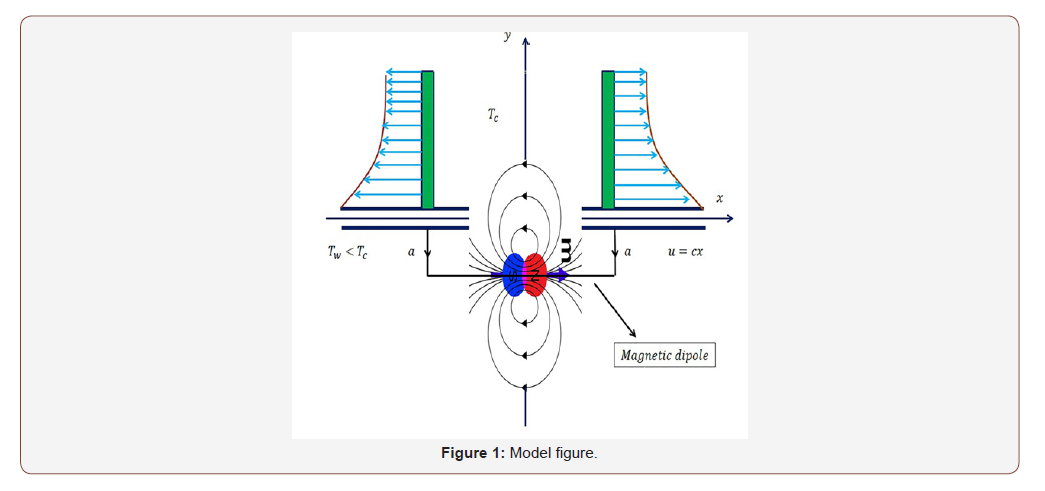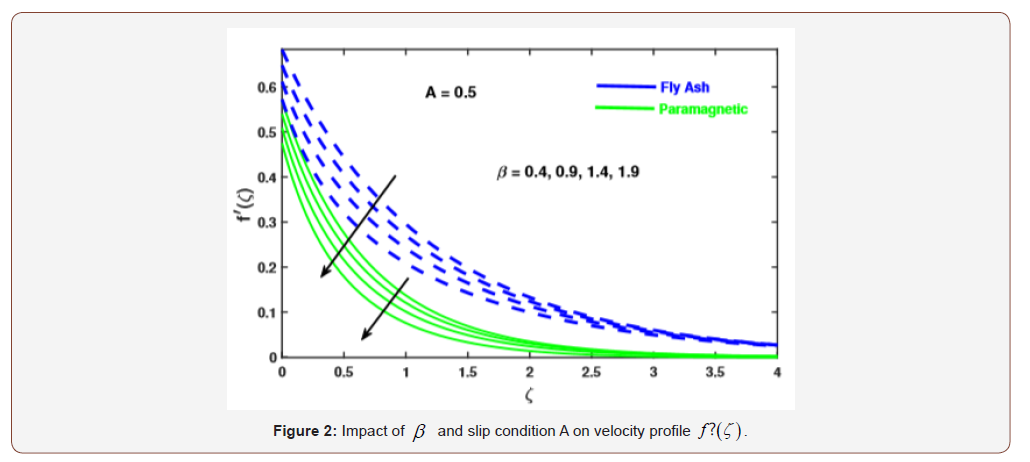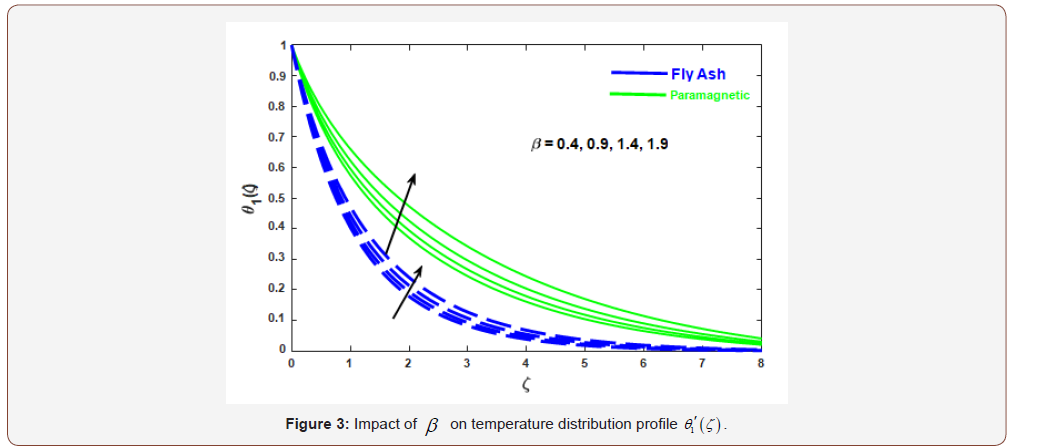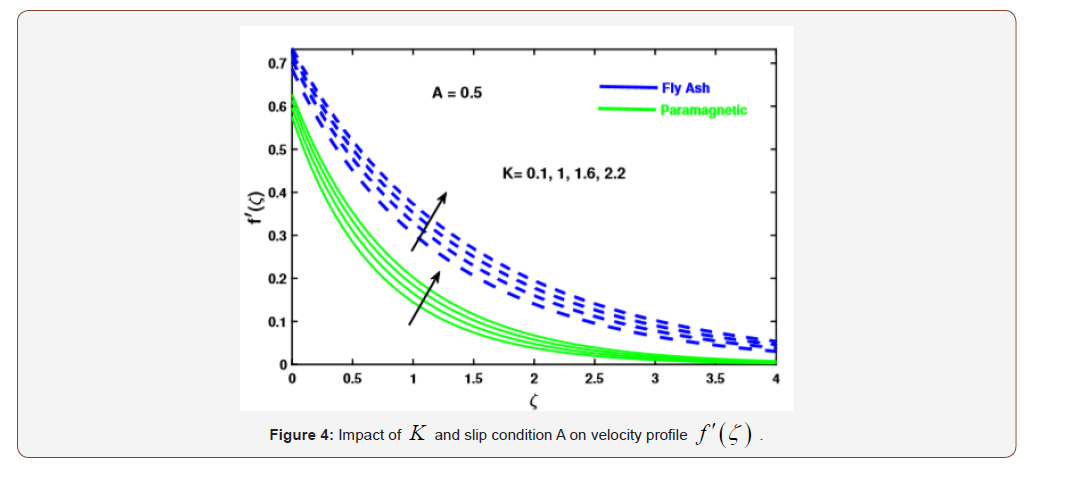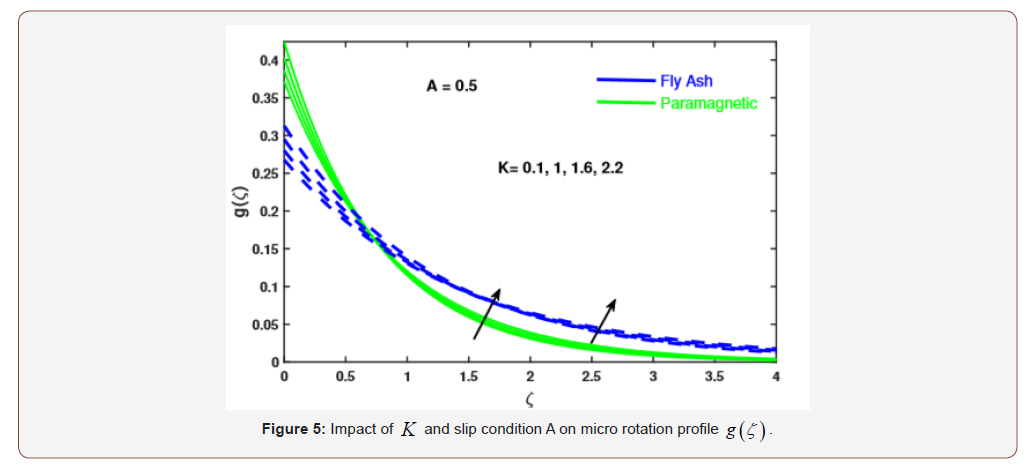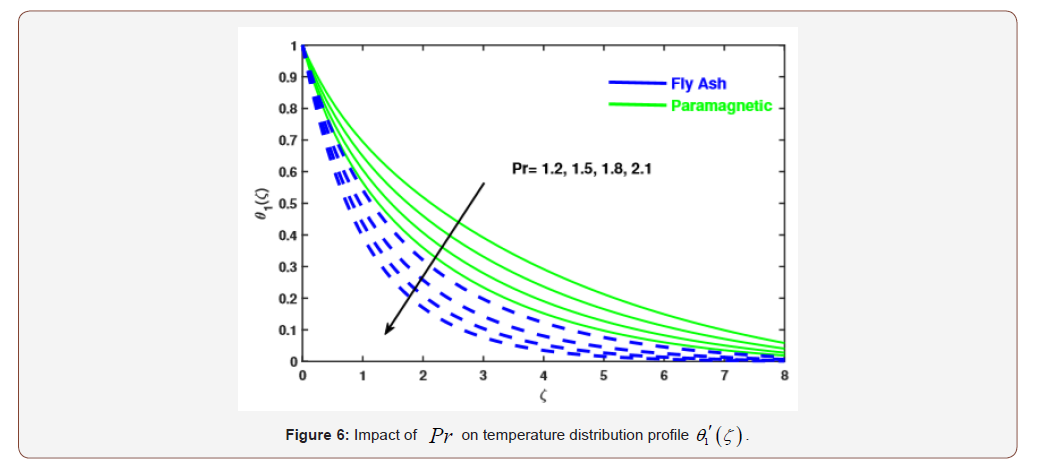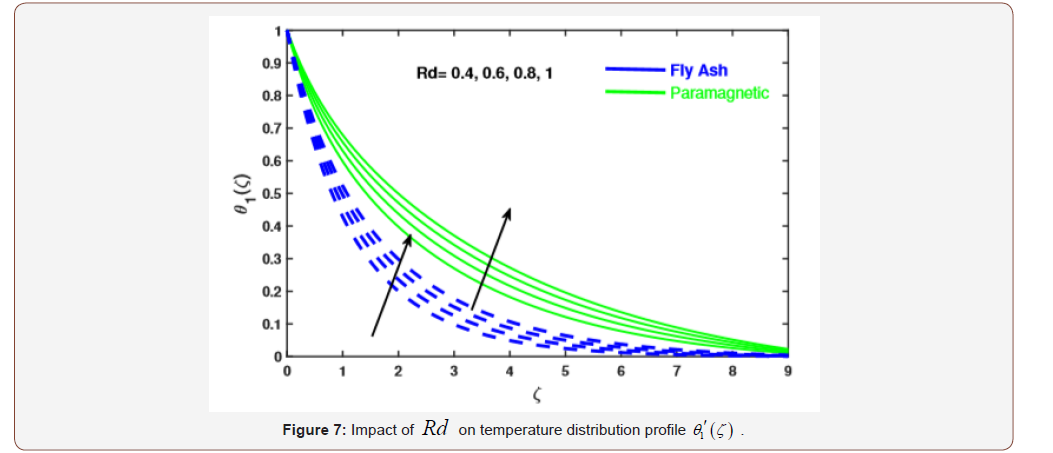Table 1:Thermo physical properties of fly ash and paramagnetic nanoparticles with water fluid.Table 2:FEM convergence results of h(ζ), g(ζ), θ1(ζ), and θ2(ζ) at the 3 of computational domain [0,10] for different number of elements when Pr = 2, K = 0.1, λ = 0.01, φ = 0.2, β = 0.5, g = 2, m = 0.5, fw = 0.2, Rd = 0.5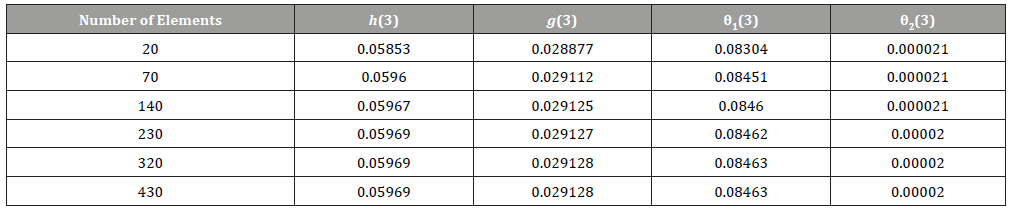Table 3:Comparison of the exact solution of Crane  and FEM for the flow velocity f ˊ(η).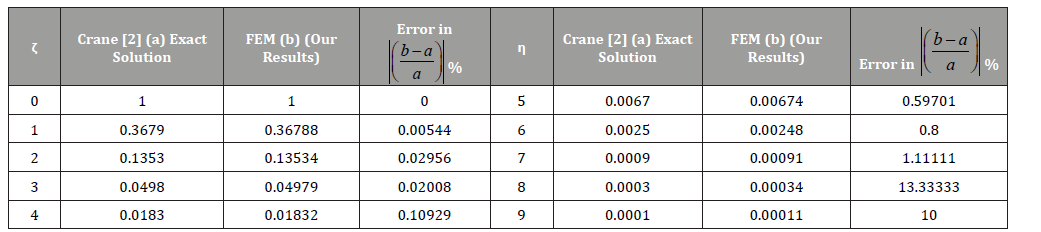Table 4:Comparison of −θˊ(0) for various values of Pr and σ when all others parameters are zero.#### Conclusion

In this study, analysis of fly ash and paramagnetic nanoparticles due to applied magnetic dipole over a stretching sheet with momentum slip condition are examined. Governing equations were solved by finite element method. The impact of relevant parameters on velocity, micro rotation velocity, and temperature are demonstrated graphically. Results revealed that.

• The velocity profile decreased and temperature profile increased with increasing ferromagnetic parameter (β ) , and also noted that the velocity boundary layer thickness for fly ash nanoparticles is much higher than that of paramagnetic ( Ta ) a T nanoparticles.

• The escalating values of K upsurges the micro rotation and velocity profile and with the presence of slip condition, the boundary layers of velocity and micro rotation profiles increases.

• The increasing values of Pr and λ reduces the temperature profile (θ1) , while the escalating values of Rd increase θ1 .

• The ferrofluid heat transfer rate (θ2) escalates with enhancing the values of λ and s.

• From the above results, we noticed that the thermal boundary layers thickness for paramagnetic nanoparticles is much higher than fly ash nanoparticles.

None.

#### Conflict of Interest

No conflict of interest.

Article Details
Citation
Keywords
Scroll to
Scroll to Top